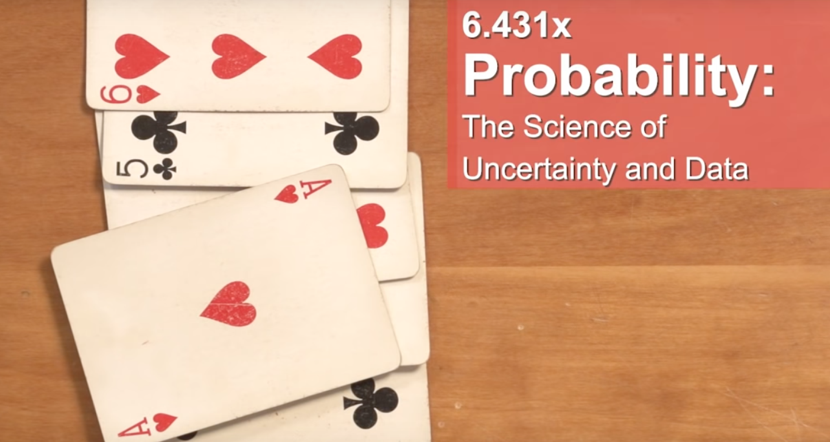Publish at March 15 2022 Updated March 18 2022

# Probability - The Science of Uncertainty and Data

## Probabilistic modeling and the related field of statistical inference are the keys to make scientifically sound predictions.The world is full of uncertainty: accidents, storms, unruly financial markets, noisy communications. The world is also full of data. Probabilistic modeling and the related field of statistical inference are the keys to analyzing data and making scientifically sound predictions.

Probabilistic models use the language of mathematics. But instead of relying on the traditional "theorem-proof" format, we develop the material in an intuitive -- but still rigorous and mathematically-precise -- manner. Furthermore, while the applications are multiple and evident, we emphasize the basic concepts and methodologies that are universally applicable.

The course covers all of the basic probability concepts, including:

• multiple discrete or continuous random variables, expectations, and conditional distributions
• laws of large numbers
• the main tools of Bayesian inference methods
• an introduction to random processes (Poisson processes and Markov chains)

The contents of this courseare heavily based upon the corresponding MIT class -- Introduction to Probability -- a course that has been offered and continuously refined over more than 50 years. It is a challenging class but will enable you to apply the tools of probability theory to real-world applications or to your research.

What you'll learn

• The basic structure and elements of probabilistic models
• Random variables, their distributions, means, and variances
• Probabilistic calculations
• Inference methods
• Laws of large numbers and their applications
• Random processes

Syllabus

Unit 1 : Probability models and axioms

• Probability models and axioms
• Mathematical background : Sets; sequences, limits, and series; (un)countable sets.

Unit 2 : Conditioning and independance

• Conditioning and Bayes' rule
• Independence

Unit 3 : Counting

Unit 4 : Discrete random variables

• Probability mass functions and expectations
• Variance; Conditioning on an event; Multiple random variables
• Conditioning on a random variable; Independence of random variables

Unit 5 : Continuous random variables

• Probability density functions
• Conditioning on an event; Multiple random variables
• Conditioning on a random variable; Independence; Bayes' rule

Unit 6 : Further topics on random variables

• Derived distributions
• Sums of independent random variables; Covariance and correlation
• Conditional expectation and variance revisited; Sum of a random number of independant random variables

Unit 7 : Bayesian inference

• Introduction to Bayesian inference
• Linear models with normal noise
• Least mean squares (LMS) estimation
• Linear least mean squares (LLMS) estimation

Unit 8 : Limit Theorems and classical statistics

• Inequalities, convergence, and the Weak Law of Large Numbers
• The Central Limit Theorem (CLT)
• An introduction to classical statistics

Unit 9 : Bernoulli and Poisson processes

Unit 10 (Optional) : Markov chains

• Finite-state Markov chains
• Steady-state behavior of Markov chains
• Absorption probabilities and expected time to absorption

### Files

• In the fog

_____________________________

_____________________________• The lessons
• The learning resources
• The file of the week
• The events
• The technologies

In addition, index your favorite resources in your own folders and find your history of consultation.

## Add to my playlists

Receive our news by email

Every day, stay informed about digital learning in all its forms. Great ideas and resources. Take advantage, it's free!